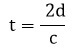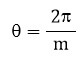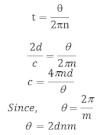# Velocity Of Light By Michelson Method

The experimental arrangement to determine the velocity of light by Michelson method is as shown in figure. It consists of three mirrors such as octagonal mirror (m1), concave mirror (m2) & plane mirror (m3). Light from the source (s) incident at an angle of 450 in one of faces of octagonal mirror (m1). The reflected light from this face falls on a distant concave mirror (m2).
With the help of plane mirror (m3) placed at a center of curvature of concave mirror (m2), the light is returned back and falls on the face of mirror (m1) again at angle of 450. The light reflected from this face is collected by telescope and observed by eye.
When the mirror (m1) is rotated, the light returning from the mirror (m1) will not in general incident at an angle 450 and not observed by eye. When rotation of mirror (m1) is so adjusted that, the face bc of mirror occupies exactly the same position as was occupied by face cd during the time light travels from m1 to m2 and returning back to m1. Then image of source will be reappear.
If ‘d’ be the distance between mirror (m1) and (m2) and ‘c’ be the velocity of light, then time taken by light to travel from m1 to m2 and returning back to m1 is given by,if ‘m’ be the no of faces of faces of polygonal mirror and ‘n’ be the no of revolution per second. Then angle rotated by mirror during the time t i.e.and time taken by mirror to rotate by angle θ i.e.In Michelson original experiment, the speed rotating octagonal mirror is about 500 rev/sec and ‘d’ is about 3.5 km. He obtained the value of c = 2.99797 × 108 m/sec in vacuum.